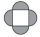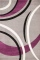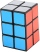# Square root + area - math problems

#### Number of problems found: 107

• SemicircleThe ornament consists of one square and four dark semicircles. The area of the square is 4 cm ^ 2. Find the area of one dark semicircle and round the result to hundreds.
• Suppose 4Suppose that 14% of all steel shafts produced by a certain process are nonconforming but can be reworked (rather than having to be scrapped). Consider a random sample of 200 shafts, and let X denote the number among these that are nonconforming and can be
• MohammedMohammed grew a garden last year. His plot of land was 6 3/4 feet by 10 feet. He wants to have twice as much area and his garden this year. How much area will the new garden take up?
• LouisLouis wants to carpet the rectangular floor of his basement. The basement has an area of 5,120 square feet. The width of the basement is 4/5 its length. What is the length of Louis's basement?
• How toHow to find a total surface of a rectangular pyramid if each face is to be 8 dm high and the base is 10 dm by 6 dm.
• Regular square prismThe volume of a regular square prism is 192 cm3. The size of its base edge and the body height is 1: 3. Calculate the surface of the prism.
• Cube-shaped boxThe cube-shaped box is filled to the brim with 2 liters of milk. Calculate the edge and surface of the box.
• Regular 4-sided pyramidFind the area (surface area) of a regular 4-sided pyramid if its height is 20 m and the wall height is 23 m.
• Squares above sidesIn a right triangle, the areas of the squares above its sides are 169; 25 and 144. The length of its longer leg is:
• Total areaCalculate the total area (surface and bases) of a prism whose base is a rhombus which diagonals of 12cm and 18cm and prism height are 10 cm.
• Cuboid edgesThe lengths of the cuboid edges are in the ratio 2: 3: 4. Find their length if you know that the surface of the cuboid is 468 m2.
• The cubeThe cube has a surface of 600 cm2. What is its volume?
• Cube V2SThe volume of the cube is 27 dm cubic. Calculate the surface of the cube.
• Extending square gardenMrs. Petrová's garden had the shape of a square with a side length of 15 m. After its enlargement by 64 m2 (square), it had the shape of a square again. How many meters has the length of each side of the garden been extended?
• Triangular prismThe triangular prism has a base in the shape of a right triangle, the legs of which is 9 cm and 40 cm long. The height of the prism is 20 cm. What is its volume cm3? And the surface cm2?
• Cube surfce2volumeCalculate the volume of the cube if its surface is 150 cm2.
• Cuboid to cubeA cuboid with dimensions of 9 cm, 6 cm, and 4 cm has the same volume as a cube. Calculate the surface of this cube.
• The cubeThe cube has a surface area of 216 dm2. Calculate: a) the content of one wall, b) edge length, c) cube volume.
• Magnified cubeIf the lengths of the cube's edges are extended by 5 cm, its volume will increase by 485 cm3. Determine the surface of both the original and the magnified cube.
• Wallpaper3750 cm square of wallpaper is needed to glue a cube-shaped box. Can Dad cut out the whole necessary piece of wallpaper as a whole if he has a roll of wallpaper 50 cm wide?

Do you have an exciting math question or word problem that you can't solve? Ask a question or post a math problem, and we can try to solve it.

We will send a solution to your e-mail address. Solved examples are also published here. Please enter the e-mail correctly and check whether you don't have a full mailbox.

Please do not submit problems from current active competitions such as Mathematical Olympiad, correspondence seminars etc...

Square root - math problems. Area - math problems.